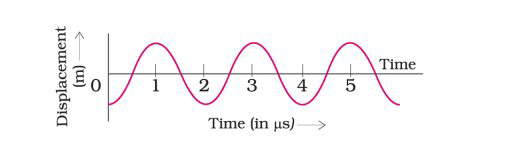# NCERT Exemplar Solutions Class 9 Science Solutions for Sound - Exercise in Chapter 12 - Sound

The given graph (Fig.12.2) shows the displacement versus time relation for a disturbance traveling with a velocity of 1500 m s–1. Calculate the wavelength of the disturbance.T= 2×106 s

Frequency v=1/𝑇 = 105hz

Wavelength λ𝑣/𝑉 =5×105m

Video transcript
"hello everyone welcome to leader learning i am good preet your science tutor today's question is the given graph figure 1 12.2 shows the displacement versus time relation for a disturbance traveling with a velocity of 1500 meter per second calculate the wavelength of the disturbance so in this question from the given graph we are going to calculate the wavelength of the disturbance so lets start our answer if you observe this graph from the graph we can see this one cycle is completed at 2 microseconds so the time period here will be 2 micro seconds and if you write it in seconds then it will be 2 into 10 to the power minus 6 seconds okay and velocity is already given that is 1500 meter per second now what we have to calculate is wavelength wavelength that is lambda can be calculated by the formula v into t where v is already given that is 1500 into time period that is 2 into 10 to the power minus 6 so from here we'll get the value of lambda as 3 into 10 to the power minus 3 meters so this will be the wavelength of the disturbance for more such videos please subscribe leader learning and for any doubts drop a comment thank you"
Related Questions
Exercises

Lido

Courses

Teachers

Book a Demo with us

Syllabus

Maths
CBSE
Maths
ICSE
Science
CBSE

Science
ICSE
English
CBSE
English
ICSE
Coding

Terms & Policies

Selina Question Bank

Maths
Physics
Biology

Allied Question Bank

Chemistry
Connect with us on social media!